## 拉普拉斯矩阵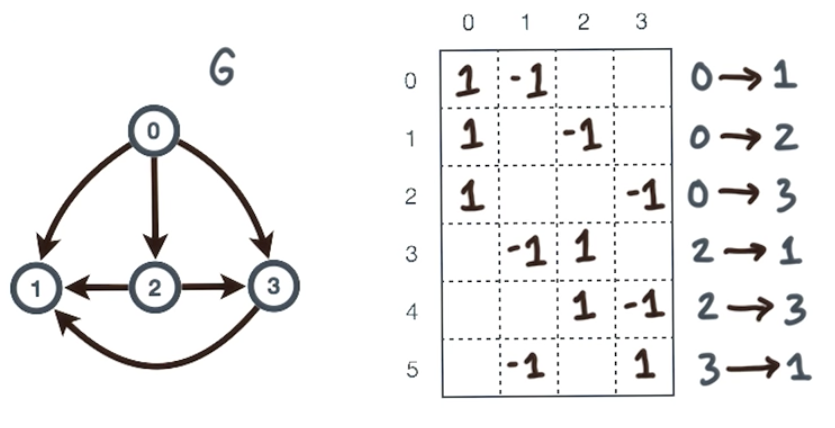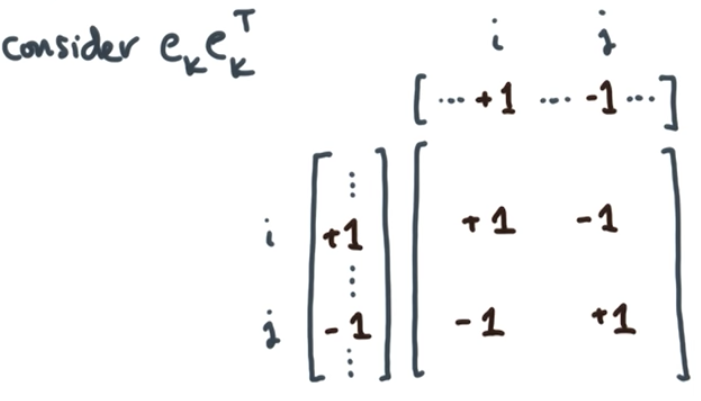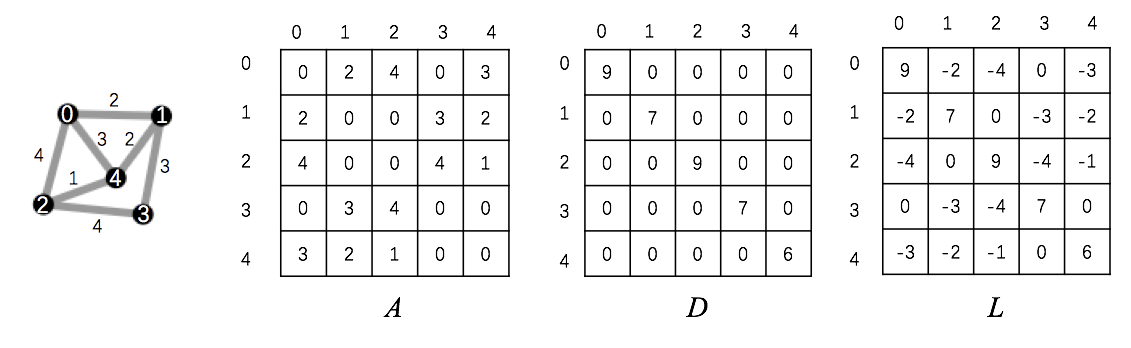## 弹簧模型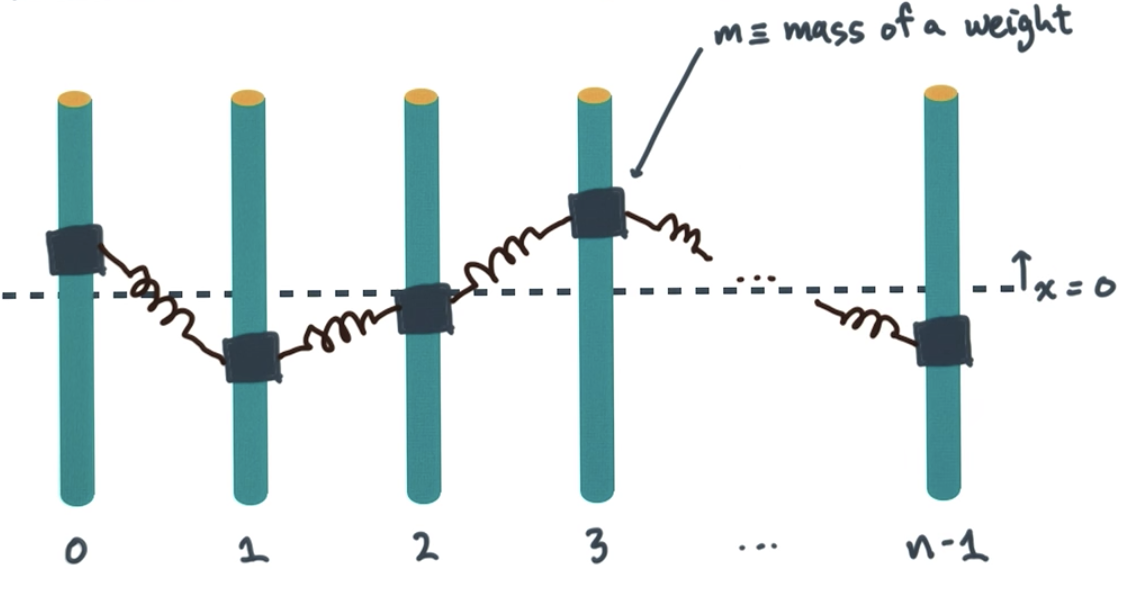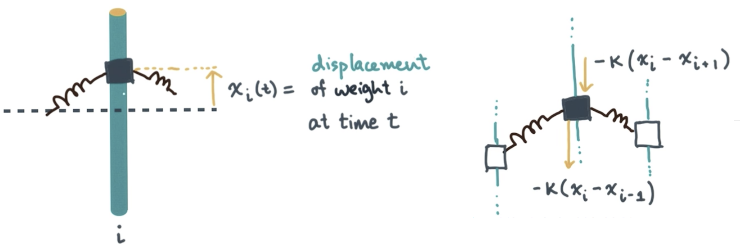## 代数连通性

1. 拉普拉斯矩阵是对称的。

2. 拉普拉斯矩阵的特征值都是实数并且非负的，而且对应的特征向量都是实数并且正交的。按照惯例，会对这些特征值进行排序：$\lambda_{n-1} \geq \lambda_{n-2} \geq \cdots \geq \lambda_0 \geq 0$

3. 如果$G$有$k$个连通子图，当且仅当：

（这一点也说明了，拉普拉斯矩阵的特征值和图的连通性有一定关联）

4. 对于一个有$n$个点的图$G$，将该图分成正负两部分。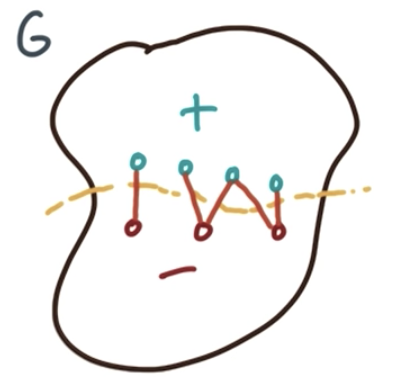记：

那么，连通+-两部分的那些切割边的数量，则可以用$\frac{1}{4} x^TL(G)x​$表示。

## Courant minimax principle

1. 创建$G$的拉普拉斯矩阵$L(G)$

2. 计算该矩阵的第二个特征向量$(\lambda_1, \vec{q}_1)$

3. 用该特征向量的正负来表达$x$，就能得到想要的分割方式。也即：